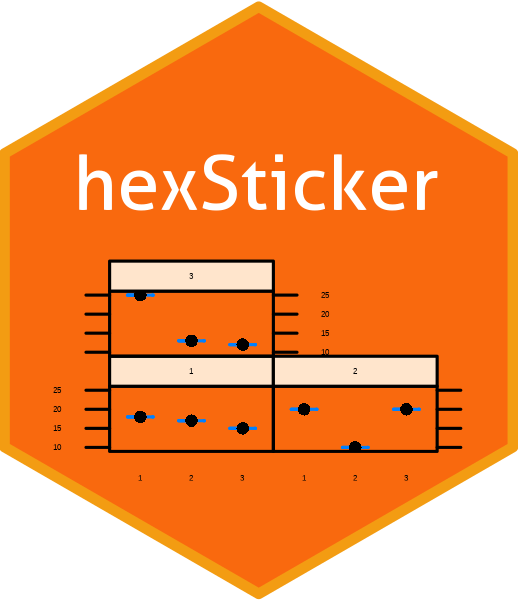## Intro

A couple of days ago, the `hexSticker` package was published on CRAN. The package provides some functions to plot hexagon stickers that may be used to promote `R` packages. As described on GitHub, the stickers can be plotted either using base `R's` plotting function, the `lattice` package or the `ggplot2` package. Moreover, it is also possible to plot image files.Since I found it quite demanding to install the `hexSticker` package on a current Linux os (Linux Mint 18.1), I decided to write a short tutorial explaining how to install and use the package on Linux Ubuntu-based operating systems.

## Linux packages required

In a first step, we need to open the terminal to install the following software packages. While `texinfo` is required to build `R` packages from source, `libudunits2-dev`, `fftw-dev` and `mffm-fftw1` are needed to install some `R` packages the `hexSticker` package depends on (`ggforce`, `fftwtools`).

``````sudo apt-get install texinfo libudunits2-dev fftw-dev mffm-fftw1 libfftw3-dev libtiff5-dev
``````

## R packages required

Recently, the `fftwtools` package was added to CRAN. Thus, it can be installed the usual way:

``````installed.packages('fftwtools', dep = TRUE)
``````

Finally, the `EBImage` package must be installed from the Bioconductor repository and the packages `ggimage`, `ggforce` and `hexSticker` must be installed from CRAN.

``````source("https://bioconductor.org/biocLite.R")
biocLite("EBImage")
install.packages("ggimage")
install.packages("ggforce")
install.packages("hexSticker")
``````

For plotting an example hexsticker we need some data provided by the `streetsofle` package which must be installed from GitHub:

``````if (!require("devtools")) install.packages("devtools")
devtools::install_github("nrkoehler/streetsofle")
``````

## Plotting

With the following code chunk we create two hexstickers for the `streetsofle` package. The colours chosen stem from the city flag of Leipzig. The arguments of the `sticker()` function are explained within the code's comments.

``````library(hexSticker)
library(ggplot2)
library(streetsofle)
data(streetsofle)

p.1 <- ggplot(aes(x = lon, y = lat), data = shape.ortsteile) +
theme_map_le() +
coord_quickmap() +
geom_polygon(aes(x = lon, y = lat, group = group),
fill = NA,
size = 0.2,
color = "#FFCB00") +
geom_polygon(aes(x = lon, y = lat, group = group),
color = "#FFCB00",
size = 1,
fill = NA,
data = shape.bezirke)

p.1 <- sticker(p.1,
package="streetsofle",
s_x = 1, # horizontal position of subplot
s_y = 1.1, # vertical position of subplot
s_width = 1.4, # width of subplot
s_height = 1.4, # height of subplot
p_x = 1, # horizontal position of font
p_y = .43, # vertical position of font
p_size = 6, # font size
p_color = "#FFCB00", # font colour
h_size = 3, # hexagon border size
h_fill = "#004CFF", # hexagon fill colour
h_color = "#FFCB00") # hexagon border colour

p.2 <- ggplot(aes(x = lon, y = lat), data = shape.ortsteile) +
theme_map_le() +
coord_quickmap() +
geom_polygon(aes(x = lon, y = lat, group = group),
fill = NA,
size = 0.2,
color = "#004CFF") +
geom_polygon(aes(x = lon, y = lat, group = group),
color = "#004CFF",
size = 1,
fill = NA,
data = shape.bezirke)

p.2 <- sticker(p.2,
package="streetsofle",
s_x = 1, # horizontal position of subplot
s_y = 1.1, # vertical position of subplot
s_width = 1.4, # width of subplot
s_height = 1.4, # height of subplot
p_x = 1, # horizontal position of font
p_y = .43, # vertical position of font
p_size = 6, # font size
p_color = "#004CFF", # font color
h_size = 3, # hexagon border size
h_fill = "#FFCB00", # hexagon fill colour
h_color = "#004CFF") # hexagon border colour
``````

Finally, both plots are put into a grid layout using the `grid.arrange()` function of the `gridExtra` package.

``````library(gridExtra)
grid.arrange(p.1, p.2, ncol = 2, respect = TRUE)
``````I'm not sure which sticker looks better. What do you think?